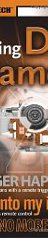:Add Comment |Related Links |TrackBackRelated Content

# Teleconverter Magnification Comparison

A posible reason is that Sigma might be basing their converter rating on image area, while Olympus might have used a focal length multiplier. A doubled focal length makes lines appear to be twice as long, but areas four times as large. Areas are a square of linear dimensions, and it just so happens that 2, the Sigma rating, is the square of 1.4, the Olympus rating.

For example, let's say you took a picture of a 2"x2" cube with a digital camera using a 50mm (equivalent) focal length. Let's assume the sides of the cubes are 400 pixels long, so the surface area of one side is 400*400=160000 pixels. A focal length multiplier of 1.4 gives us 70mm, and sides of 560 pixels. Squaring that we get 313600 pixels for area, pretty close to twice of 160000.

Andrea Reina
Thu, 29 Dec 2005 09:23:10 -0800Add Comment |Related Links |TrackBackRelated ContentSpam Control | * indicates required fieldSend Ping |TrackBack URL |Spam Control